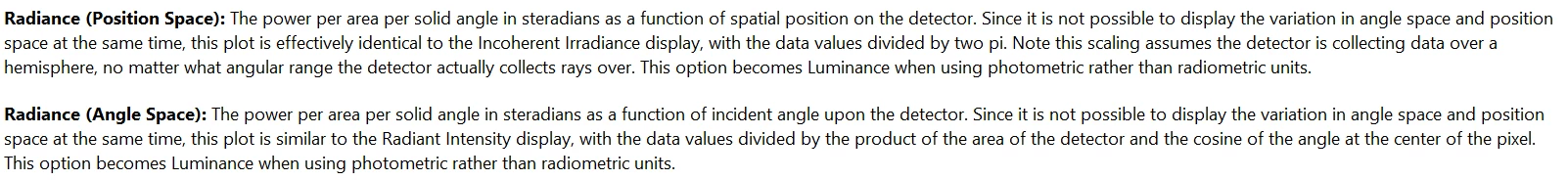# Radiance: Angle Space vs. Position Space

• 3 replies
• 524 views• Monochrome
• 2 replies

My NS-system comprises 2 components only.

1. Source Rectangle x/y Half Width = 5mm; Cosine Exponent = 1; Power = 1W

This is nothing else than a pure Lambertian emitter.

2. Detector Rectangle x/y Half Width = 5mm; 100x100 pixel; 0.001 mm behind the source

This detector covers closely the Lambertian Emitter

The Power (P) of a Lambertian source calculates as:

P = pi * A * NA^2 * L

A: Area of source

NA: Numerical aperture of source; NA = 1 for hemisphere

For L we get:

L = P / (pi * A * NA^2); P = 1W; NA = 1; A = 1cm^2

L = 1 / pi = 0.318

Ratracing confirms this calculation for radiance in angle space:Of course there is a very little amount of noise...

When I switch the detector from Radiance in angle space to Radiance position space, the result gets wrong by a factor of 2:Now I am really puzzled: A pure Lambertian source has a constant radiance in both, angle space and position space. Basically, the raytracing confirms this.

However, the radiance also needs the same numerical value in both projections. That is the nature of a constant.

The Zemax file is so simple that your engineers can set it up in seconds. The raytracing itself should not take long as well.

Kind regards,

Joachim

### 3 replies

Userlevel 1Hello Joachim,

This distinction may come from the way that radiance is calculated in OpticStudio.Radiance in position space is effectively the Incoherent Irradiance divided by 2 pi while the Radiance in angle space is the Radiant Intesnity divided by the product of the area of the detector and cosine of the angle at the center of the pixel.

For reference, these definitions are found in the Help System under the 'Detector Viewer' page.Hello Kaleb,

Yes, in the general case the radiance 'L' is a scalar function of four variables, i.e. 'x', 'y,', 'u', 'v'. The latter two are the corresponsing cosine of direction.

L = L(x,y,u,v)

(Of course u and v can easily be expressed and displayed also in degrees [°].)

A very special and the easiest case is a Lambertian source. Here the radiance is not a function of the named 4 variables, it is just a constant.

L = const. (= P/(pi * A)

If the ZEMAX detector reports two different numbers for this constant, there are to possible interpretations in my view:

1. The two different detector views should not be named Radiance (in angle/position space) for they are something different. (btw, Radiance in angle space is correct, while position space is not.)

2. It is just a classical bug.

Now I hope for some resonance in the forum community. In the best case my reply triggers an internal discussion of the ZEMAX experts.

Thank you again, kind regards,

Joachim

Userlevel 4+1

Hi Joachim,

Thank you for your post! Since you also sent an email to support inbox so I replied to you via email and wonder if you have received it.

As Kaleb posted above, the Help File at The Analyze Tab (non-sequential ui mode) > Detectors Group > Detector Viewer provides a description on how OpticStudio computes the 'Radaince (Position Space)' and 'Radiance (Angle Space)'. I agree that this is not the textbook definition of Radiance which should be a function of both angle and position simultaneoulsy. This is basically due to the limitation of displaying 3D data in a 2D plot, that OpticStudio cannot display the variation in angle space and position space at the same time.

That said, I agree this could potentially cause confusition if the customer is not aware of that's how OpticStudio computes the Radiance (Position Space) and Radiance (Angle Space). I will discuss this with my colleagues to see if there is a better way of labelling this data in the Detector Viewer. Also, any comments or feedbacks in the forum from our users are welcome!

Best regards,

Hui